0# Change

How can change number to text. For example amount in invoice is 5000usd . I will see text too in down invoice. Five thousand dollars

68replies Oldest first
• Oldest first
• Popular•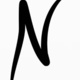• Nick
• Nick
• 4 yrs ago
• Reported - view

Hi Sean,

take a look at the number settings: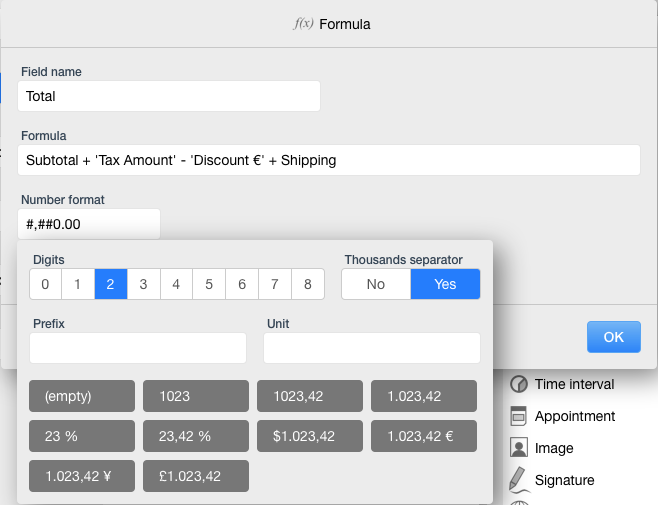...and more common for plural is Euros.

Screenshots are from Mac app version of Ninox.

Nick

Like
•• Nick
• Nick
• 4 yrs ago
• Reported - view

...and the Mac Settings: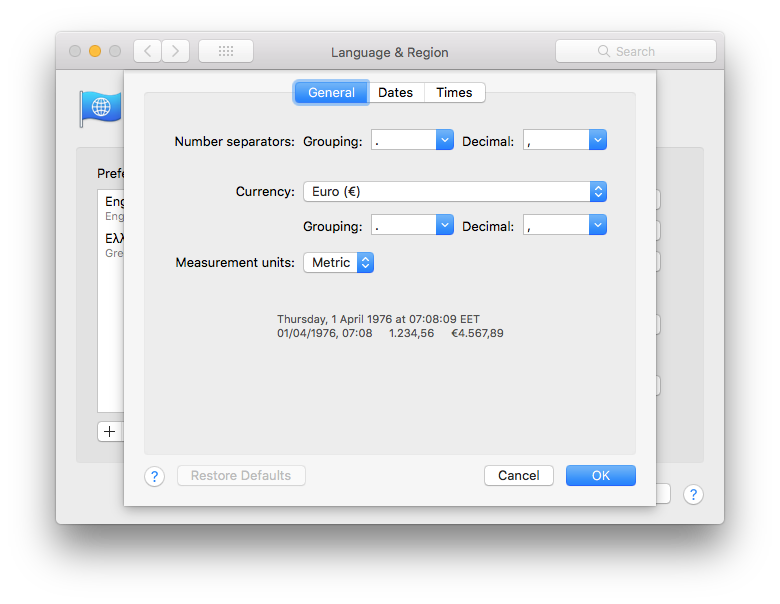Nick

Like
•• Sean
• Sean
• 4 yrs ago
• Reported - view

Nick, thanks for the screenshots. When I saw the system settings screenshot, I wanted to facepalm! It is a simple fix on these 2 lines:

`if contains(text(numAmount), ".") then`
`let dpIndex := index(text(numAmount), ".");`

If you change "." to "," it should work correctly. I did change the system settings on my Mac and it tested correctly. The decimal separator just needs to be unique for the code to work as it is written.

Like
•• Sean
• Sean
• 4 yrs ago
• Reported - view

This is the complete formula. It checks for single and plural amounts and adds "and no cents" if there isn't any change. I'll post the same formula without the "and no cents" after this.

`let empty := "";`
`let x := [empty, "One ", "Two ", "Three ", "Four ", "Five ", "Six ", "Seven ", "Eight ", "Nine ", "Ten ", "Eleven ", "Twelve ", "Thirteen ", "Fourteen ", "Fifteen ", "Sixteen ", "Seventeen ", "Eighteen ", "Nineteen "];`
`let y := [empty, empty, "Twenty ", "Thirty ", "Forty ", "Fifty ", "Sixty ", "Seventy ", "Eighty ", "Ninety "];`
`"/*                       ";`
`" * User defined functions";`
`" */                      ";`
`function mod(dividend : number,divisor : number) do`
`dividend - divisor * floor(dividend / divisor)`
`end;`
`function numberToWord(num : number,suffix : text) do`
`if floor(num) != 0 then`
`if floor(num) > 19 then`
`item(y, floor(num / 10)) + item(x, mod(num, 10)) + suffix`
`else`
`item(x, num) + suffix`
`end`
`else`
`empty`
`end`
`end;`
`"/*             ";`
`" * Main program";`
`" */            ";`
`"// Number to be converted to words";`
`let numAmount := Net;`
`"// Test for number of digits";`
`if length(format(floor(numAmount), "0")) < 11 then`
`"// String to store word representation of given number";`
`let NtW := "";`
`"// This handles Cents";`
`if contains(text(numAmount), ",") then`
`let dpIndex := index(text(numAmount), ",");`
`let numCents := number(substr(text(numAmount), dpIndex + 1, 2));`
`if floor(numAmount) > 0 then NtW := "and " end;`
`if numCents > 0 then`
`if numCents > 1 then`
`NtW := NtW + numberToWord(mod(numCents, 100), "Cents")`
`else`
`NtW := NtW + numberToWord(mod(numCents, 100), "Cent")`
`end`
`else`
`if floor(numAmount) > 0 then`
`NtW := NtW + "no Cents"`
`else`
`NtW := ""`
`end`
`end`
`end;`
`"// This code uses Euro for both singular and plural";`
`"// if floor(numAmount) > 0 then NtW := Euro  + NtW end;";`
`if floor(numAmount) > 0 then`
`if floor(numAmount) > 1 then`
`NtW := "Euros " + NtW`
`else`
`NtW := "Euro " + NtW`
`end`
`end;`
`"// This handles digits at Ones and Tens place";`
`NtW := numberToWord(mod(numAmount, 100), "") + NtW;`
`"// This handles digit at Hundreds place";`
`NtW := numberToWord(mod(numAmount / 100, 10), "Hundred ") + NtW;`
`"// This handles digits at One Thousands and Ten Thousands place";`
`NtW := numberToWord(mod(numAmount / 1000, 100), "Thousand ") + NtW;`
`"// This handles digit at Hundred Thousands place";`
`NtW := numberToWord(mod(numAmount / 100000, 10), "Hundred ") + NtW;`
`"// This handles digits at One Millions and Ten Millions place";`
`NtW := numberToWord(mod(numAmount / 1000000, 100), "Million ") + NtW;`
`"// This handles digit at Hundred Millions place";`
`NtW := numberToWord(mod(numAmount / 100000000, 10), "Hundred ") + NtW;`
`"// This handles digit at One Billions place";`
`NtW := numberToWord(mod(numAmount / 1000000000, 10), "Billion ") + NtW;`
`NtW`
`else`
`"Number is too large"`
`end`

Like
•• Sean
• Sean
• 4 yrs ago
• Reported - view

This just describes the whole Euro amounts without the "and no Cents".

`let empty := "";`
`let x := [empty, "One ", "Two ", "Three ", "Four ", "Five ", "Six ", "Seven ", "Eight ", "Nine ", "Ten ", "Eleven ", "Twelve ", "Thirteen ", "Fourteen ", "Fifteen ", "Sixteen ", "Seventeen ", "Eighteen ", "Nineteen "];`
`let y := [empty, empty, "Twenty ", "Thirty ", "Forty ", "Fifty ", "Sixty ", "Seventy ", "Eighty ", "Ninety "];`
`"/*                       ";`
`" * User defined functions";`
`" */                      ";`
`function mod(dividend : number,divisor : number) do`
`dividend - divisor * floor(dividend / divisor)`
`end;`
`function numberToWord(num : number,suffix : text) do`
`if floor(num) != 0 then`
`if floor(num) > 19 then`
`item(y, floor(num / 10)) + item(x, mod(num, 10)) + suffix`
`else`
`item(x, num) + suffix`
`end`
`else`
`empty`
`end`
`end;`
`"/*             ";`
`" * Main program";`
`" */            ";`
`"// Number to be converted to words";`
`let numAmount := Net;`
`"// Test for number of digits";`
`if length(format(floor(numAmount), "0")) < 11 then`
`"// String to store word representation of given number";`
`let NtW := "";`
`"// This handles Cents";`
`if contains(text(numAmount), ",") then`
`let dpIndex := index(text(numAmount), ",");`
`let numCents := number(substr(text(numAmount), dpIndex + 1, 2));`
`if floor(numAmount) > 0 then NtW := "and " end;`
`if numCents > 0 then`
`if numCents > 1 then`
`NtW := NtW + numberToWord(mod(numCents, 100), "Cents")`
`else`
`NtW := NtW + numberToWord(mod(numCents, 100), "Cent")`
`end`
`else`
`NtW := ""`
`end`
`end;`
`"// This code uses Euro for both singular and plural";`
`"// if floor(numAmount) > 0 then NtW := Euro  + NtW end;";`
`if floor(numAmount) > 0 then`
`if floor(numAmount) > 1 then`
`NtW := "Euros " + NtW`
`else`
`NtW := "Euro " + NtW`
`end`
`end;`
`"// This handles digits at Ones and Tens place";`
`NtW := numberToWord(mod(numAmount, 100), "") + NtW;`
`"// This handles digit at Hundreds place";`
`NtW := numberToWord(mod(numAmount / 100, 10), "Hundred ") + NtW;`
`"// This handles digits at One Thousands and Ten Thousands place";`
`NtW := numberToWord(mod(numAmount / 1000, 100), "Thousand ") + NtW;`
`"// This handles digit at Hundred Thousands place";`
`NtW := numberToWord(mod(numAmount / 100000, 10), "Hundred ") + NtW;`
`"// This handles digits at One Millions and Ten Millions place";`
`NtW := numberToWord(mod(numAmount / 1000000, 100), "Million ") + NtW;`
`"// This handles digit at Hundred Millions place";`
`NtW := numberToWord(mod(numAmount / 100000000, 10), "Hundred ") + NtW;`
`"// This handles digit at One Billions place";`
`NtW := numberToWord(mod(numAmount / 1000000000, 10), "Billion ") + NtW;`
`NtW`
`else`
`"Number is too large"`
`end`

Like
•• Nick
• Nick
• 4 yrs ago
• Reported - view

Sean, I think you did it!

It tested correctly.

Again, thanks a million!

Nick

Like
•• Sean
• Sean
• 4 yrs ago
• Reported - view

Nick, you're welcome. There is another simple change that can expand it's usefulness if you use multiple currencies. Create a table for currencies like this: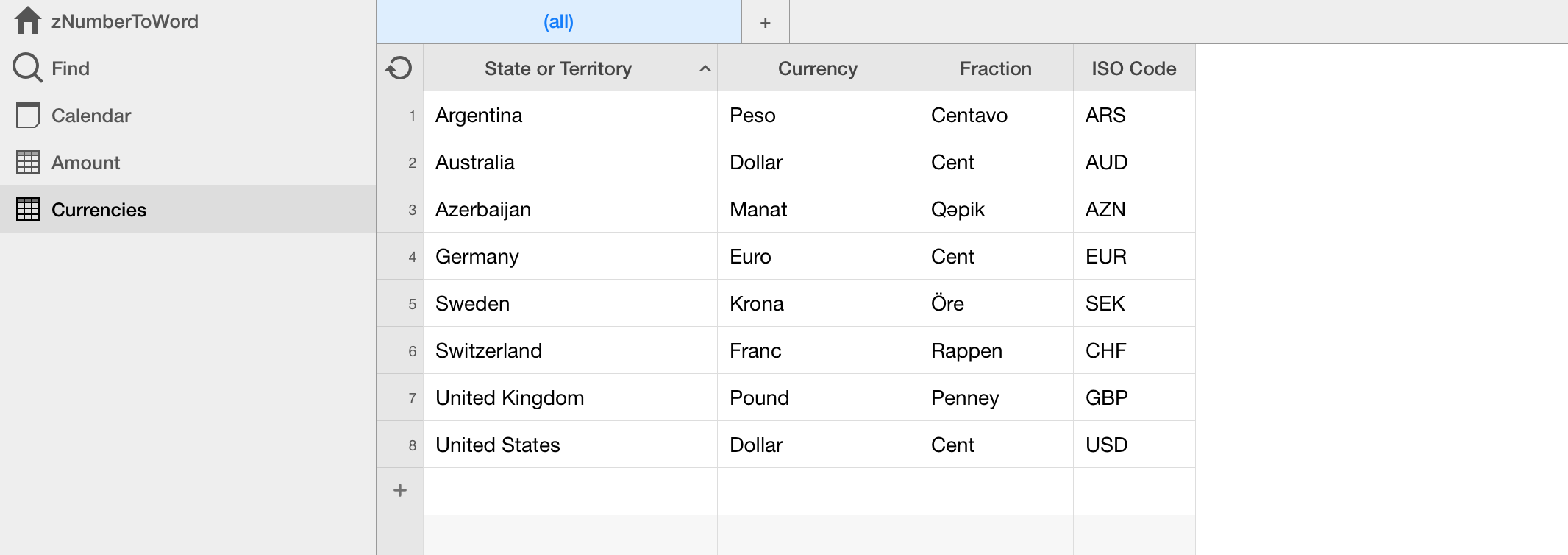Create a link in any tables where you would want to use the formula and change the code like this.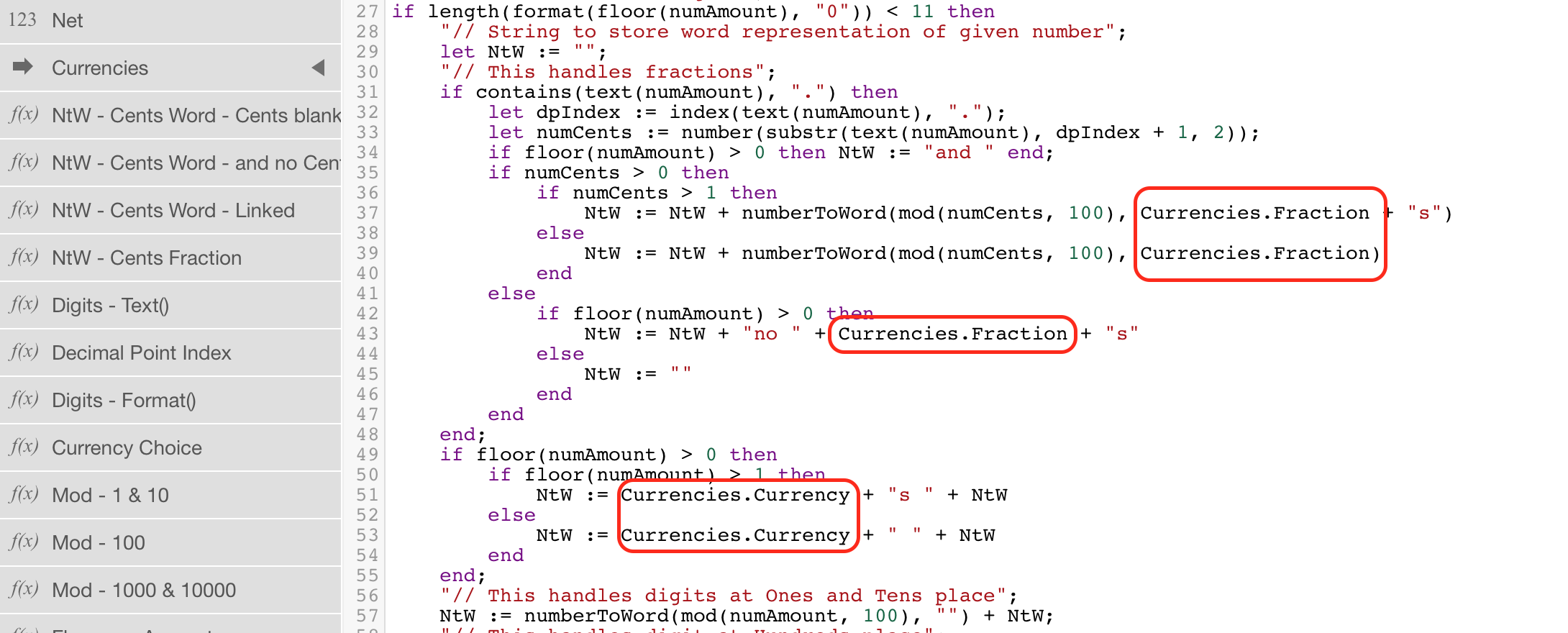Sean

Like
•Thank you very much Sean for this code that works very well.

But in French, it is just a bit different :

- You don't say "One Hundred" but you say "hundred", but you say "Two Hundred", "Three Hundred"...

- it is the same for "One Thousand" that you say "Thousand", but it's OK for "One Million" or "One Billion"

- the most difficult, in french, is "Seventy", that you say as "Sixty-Ten", "Seventy One" that you say "Sixty Elven"... "Eighty" is ok, but you say "Ninety"  as "Eighty-ten", and "Ninety Nine" as "Ninety-Nineteen".

I'm sorry to speack french, but do you think you can find a way to solve those problems ?

Regards

Olivier

Like
•• Sean
• Sean
• 3 yrs ago
• Reported - view

I assume you meant "Eighty-Nineteen" for "Ninety Nine". I found this, http://www.frenchtutorial.com/en/learn-french/counting/70-99, is it what you want?

It will take me a while to make the time to wrap my head around it.

Like
•• blackie
• blackie
• 3 yrs ago
• Reported - view

Wow. French counting is crazy.

Eighty in French is quatre-vingts.

quatre-vingts In English is four-twentys

Eighty one is quatre-vingt-un

80 blaze it

Like
•• Sean
• Sean
• 3 yrs ago
• Reported - viewMy wife heard me chuckling and she wanted to know what was up. It wasn't until I explained the background of this thread that I caught the four-twentys reference!Like
•Yes, that's what I mean... "Eighty-Nineteen" for "Ninety Nine".

Like
•Well, I absolutely needed it in french, so I fiddled this formula (if anyone wants to simplify it, welcome !). Problems of french spelling mistakes are normally ok,I think,because french is really hard with spelling numbers.

" ----------------------";
" - TABLE DES DIZAINES -";
" ----------------------";
let x := ["zéro ", "un ", "deux ", "trois ", "quatre ", "cinq ", "six ", "sept ", "huit ", "neuf ", "dix ", "onze ", "douze ", "treize ", "quatorze ", "quinze ", "seize ", "dix-sept ", "dix-huit ", "dix-neuf ", "vingt ", "vingt et un ", "vingt-deux ", "vingt-trois ", "vingt-quatre ", "vingt-cinq ", "vingt-six ", "vingt-sept ", "vingt-huit ", "vingt-neuf ", "trente ", "trente et un ", "trente-deux ", "trente-trois ", "trente-quatre ", "trente-cinq ", "trente-six ", "trente-sept ", "trente-huit ", "trente-neuf ", "quarante ", "quarante et un ", "quarante-deux ", "quarante-trois ", "quarante-quatre ", "quarante-cinq ", "quarante-six ", "quarante-sept ", "quarante-huit ", "quarante-neuf ", "cinquante ", "cinquante et un ", "cinquante-deux ", "cinquante-trois ", "cinquante-quatre ", "cinquante-cinq ", "cinquante-six ", "cinquante-sept ", "cinquante-huit ", "cinquante-neuf ", "soixante ", "soixante et un ", "soixante-deux ", "soixante-trois ", "soixante-quatre ", "soixante-cinq ", "soixante-six ", "soixante-sept ", "soixante-huit ", "soixante-neuf ", "soixante-dix ", "soixante et onze ", "soixante-douze ", "soixante-treize ", "soixante-quatorze ", "soixante-quinze ", "soixante-seize ", "soixante-dix-sept ", "soixante-dix-huit ", "soixante-dix-neuf ", "quatre-vingt ", "quatre-vingt-un ", "quatre-vingt-deux ", "quatre-vingt-trois ", "quatre-vingt-quatre ", "quatre-vingt-cinq ", "quatre-vingt-six ", "quatre-vingt-sept ", "quatre-vingt-huit ", "quatre-vingt-neuf ", "quatre-vingt-dix ", "quatre-vingt-onze ", "quatre-vingt-douze ", "quatre-vingt-treize ", "quatre-vingt-quatorze ", "quatre-vingt-quinze ", "quatre-vingt-seize ", "quatre-vingt-dix-sept ", "quatre-vingt-dix-huit ", "quatre-vingt-dix-neuf "];
void;
" --------------------------";
" - Centaine de milliards - ";
" --------------------------";
let nb_cent_mlrds := floor(En_chiffres / 100000000000);
let cent_mlrds := nb_cent_mlrds * 100000000000;
let l_cent_mlrds := if nb_cent_mlrds > 1 then
item(x, nb_cent_mlrds)
end + if nb_cent_mlrds > 0 then
if En_chiffres = cent_mlrds and nb_cent_mlrds > 1 then
"cents "
else
"cent "
end
end;
" -------------------------";
" - Dizaine de milliards - ";
" -------------------------";
let nb_diz_mlrds := floor((En_chiffres - cent_mlrds) / 1000000000);
let diz_mlrds := nb_diz_mlrds * 1000000000;
let l_diz_mlrds := if nb_diz_mlrds > 0 then
if item(x, nb_diz_mlrds) = "quatre-vingt " then
"quatre-vingts "
else
item(x, nb_diz_mlrds)
end
end + if nb_diz_mlrds > 0 or nb_cent_mlrds > 0 then
if cent_mlrds + diz_mlrds = En_chiffres and nb_cent_mlrds * 100 + nb_diz_mlrds > 1 then
"milliards d'"
else
if cent_mlrds + diz_mlrds = En_chiffres then
"milliard d'"
else
if nb_cent_mlrds * 100 + nb_diz_mlrds > 1 then
"milliards "
else
"milliard "
end
end
end
end;
" --------------------------";
" - centaines de millions - ";
" --------------------------";
let nb_cent_million := floor((En_chiffres - (cent_mlrds + diz_mlrds)) / 100000000);
let cent_million := nb_cent_million * 100000000;
let l_cent_million := if nb_cent_million > 1 then
item(x, nb_cent_million)
end + if nb_cent_million > 0 then
if En_chiffres = cent_mlrds + diz_mlrds + cent_million and nb_cent_million > 1 then
"cents "
else
"cent "
end
end;
" -------------------------";
" - dizaines de millions - ";
" -------------------------";
let nb_diz_million := floor((En_chiffres - (cent_mlrds + diz_mlrds + cent_million)) / 1000000);
let diz_million := nb_diz_million * 1000000;
let l_diz_million := if nb_diz_million > 0 then
if item(x, nb_diz_million) = "quatre-vingt " then
"quatre-vingts "
else
item(x, nb_diz_million)
end
end + if nb_diz_million > 0 or nb_cent_million > 0 then
if cent_mlrds + diz_mlrds + cent_million + diz_million = En_chiffres and nb_cent_million * 100 + nb_diz_million > 1 then
"millions d'"
else
if cent_mlrds + diz_mlrds + cent_million + diz_million = En_chiffres then
"million d'"
else
if nb_cent_million * 100 + nb_diz_million > 1 then
"millions "
else
"million "
end
end
end
end;
" -----------------------";
" - centaines de mille - ";
" -----------------------";
let nb_cent_mille := floor((En_chiffres - (cent_mlrds + diz_mlrds + cent_million + diz_million)) / 100000);
let cent_mille := nb_cent_mille * 100000;
let l_cent_mille := if nb_cent_mille > 1 then
item(x, nb_cent_mille)
end + if nb_cent_mille > 0 then "cent " end;
" ----------------------";
" - dizaines de mille - ";
" ----------------------";
let nb_diz_mille := floor((En_chiffres - (cent_mlrds + diz_mlrds + cent_million + diz_million + cent_mille)) / 1000);
let diz_mille := nb_diz_mille * 1000;
let l_diz_mille := if nb_diz_mille > 1 then
item(x, nb_diz_mille)
end + if nb_diz_mille > 0 or nb_cent_mille > 0 then
"mille "
end;
" --------------";
" - centaines - ";
" --------------";
let nb_cent := floor((En_chiffres - (cent_mlrds + diz_mlrds + cent_million + diz_million + cent_mille + diz_mille)) / 100);
let centaine := nb_cent * 100;
let l_centaine := if nb_cent > 1 then item(x, nb_cent) end + if nb_cent > 0 then
if En_chiffres = cent_mlrds + diz_mlrds + cent_million + diz_million + cent_mille + diz_mille + centaine and centaine > 1 then
"cents "
else
"cent "
end
end;
" -------------";
" - dizaines - ";
" -------------";
let nb_diz := floor(En_chiffres - (cent_mlrds + diz_mlrds + cent_million + diz_million + cent_mille + diz_mille + centaine));
let dizaine := nb_diz;
let l_dizaine := if nb_diz > 0 then
if item(x, nb_diz) = "quatre-vingt " then
"quatre-vingts "
else
item(x, nb_diz)
end
end;
" --------------";
" - décimales - ";
" --------------";
let decim := round(100 * (En_chiffres - floor(En_chiffres)), 0);
let l_decimale := if floor(En_chiffres) > 1 then
"euros"
else
"euro"
end + if decim > 0 then
" et " + item(x, decim) + if decim > 1 then "cents" else "cent" end
end;
l_cent_mlrds + l_diz_mlrds + l_cent_million + l_diz_million + l_cent_mille + l_diz_mille + l_centaine + l_dizaine + l_decimale

Like
•• Sean
• Sean
• 2 yrs ago
• Reported - view

Olivier, well done. The only thing I would do differently is use the user-defined function and you can deleted the "void;". Glad you got it working. :)

Like
•Hi Sean, I'm sorry but I'm afraid I don't understand what you mean with the user-defined function. I don't understant why "void" automatically appears.

Like
•• Sean
• Sean
• 2 yrs ago
• Reported - view

Hi Olivier, In my code there is a user-defined function named `numberToWord`. By using a function, you can reduce repeated code, but the important thing here is you've got it working. I was able to delete "void" and it didn't reappear so my *guess* is there is a variable declared in your code that isn't used so "void" is inserted.

Like
•OK, thank you Sean.

I create a user-defined function, it's really easier too use in the DB.

Like
Like Follow
• 2 yrs agoLast active
• 68Replies
• 16311Views